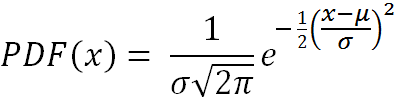# Gaussian (Normal) Random Number Generator

The Gaussian or Normal distribution is widely used in statistical tests and analysis of sample data. Most random number generators produce numbers that are uniformly distributed between a given minimum and maximum, however, researchers often need to generate sets of numbers that are normally distributed.

The tool below will generate N normally distributed random numbers when you input the mean μ and standard deviation σ.

 μ =       σ =       N = Decimal Precision integer tenths hundredths thousandths Display comma separated vertical

After the list is generated, you can copy and paste the set of numbers and analyze them using the Descriptive Statistics Calculator, which computes the mean, median, variance, standard deviation, and other statistical measures.

For a normally distributed random variable with probability density functionthe mean, median, mode, variance, s.d., and skewness are given by

mean = μ
median = μ
mode = μ
variance = σ²
s.d. = σ
skew = 0